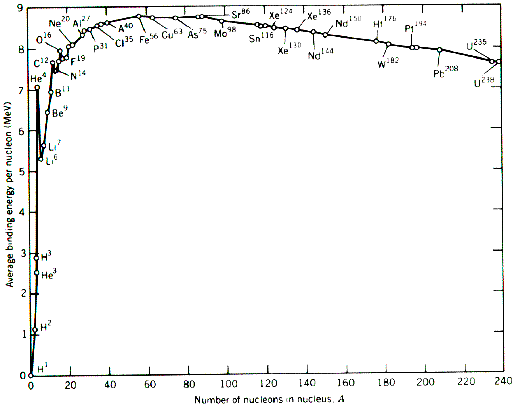QUESTION #534

# Why does the nuclear fusion reaction yield more energy than the nuclear fission reaction?

Fission only produces more energy than it consumes in large nuclei (common examples are Uranium & Plutonium, which have around 240 nucleons (nucleon = proton or neutron)). Fusion only produces more energy than it consumes in small nuclei (in stars, Hydrogen & its isotopes fusing into Helium). The energy released when 4 Hydrogen nuclei (= protons) fuse (there are some decays involved as well) into a Helium nucleus is around 27 Million Electron Volts (MeV), or about 7 MeV per nucleon.

For fission of U or P, energies released are around 200 MeV or so. The energy per event is greater (in these examples) in fission, but the energy per nucleon (fusion = about 7 MeV/nucleon, fission = about 1 Mev/nucleon) is much greater in fusion.

Fission releases the energy of the electromagnetic force when positively charged parts of the nucleus fly away from one another. Fusion releases the energy of the strong force (much stronger at short distances than the EM force) when the small pieces are captured and held into one nucleus.
Answered by: Bill Baird, Ph.D., Postdoc, College of Charleston, SC

To answer this you need to look at the binding energy per nucleon graph as follows:Now lets look at fission. An example of fission is when a Uranium-235 atom is split by a neutron into a Barium-144 atom, a Krypton-89 atom and three neutrons. Now looking at the graph the binding energy per nucleon for Uranium is about 7.6MeV and for Barium around 8.3 giving an increase in binding energy during fission of about 0.7MeV per nucleon, or a total of 164.5MeV in total. In a fusion reaction firstly two hydrogens fuse to form a deuterium (an isotope of hydrogen with nucleon no 2), a positron and an electron neutrino. Then the deuterium fuses with another hydrogen to form Helium-3 and a photon of energy. Finally two Helium-3s fuse forming a Helium nucleus and two hydron nuclei. Considering the mass of the four protons/hydrogen nuclei (4.029106u) and the mass of the Helium produced (4.002603u) we get a mass difference of 0.026503u or 24.69MeV. So it is easy to see that fusion reactions give out more energy per reaction. However, the energy per unit mass is more relevant. This is 0.7MeV for fission and 6.2MeV for fusion so it is obvious that fusion is the more effective nuclear reaction. However, you must remember that an enormous amount of energy is required in order for these reactions to occur at all - that is why fusion is not yet a practical source of energy.
Answered by: Martin Archer, Physics Student, Imperial College, London, UK